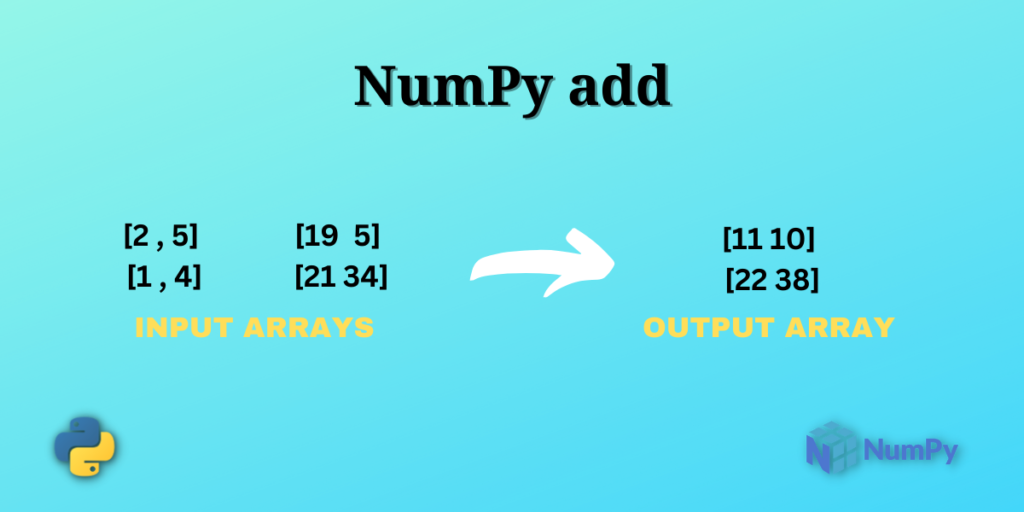# NumPy add – Explained in a Simple WayIn this article, we will be exploring the NumPy add function. Have you ever thought about how we can add two numbers or two arrays of numbers using programming? Well, that’s where the Python NumPy library comes into play.

In this tutorial, we will go through the syntax of the function and practice different types of examples as well. So, let’s get started.

`numpy.add()` is one of the mathematical functions of the NumPy library. It simply adds the values passed as input to the function.

Yes, it is that simple by definition 🙂

Let’s look at the syntax and understand more about the function.

```numpy.add(x1 , x2)
```

Here, the inputs `x1` and `x2` are NumPy array of numbers.

Note: In the syntax, the inputs can also be scalar values(simple numbers) and Python lists.

### NumPy add with Scalar Values

```# Importing the NumPy module
import numpy as np

a = 5
b = 4

print("The sum of 5 and 4 is:",np.add(a , b))
```

Output

```The sum of 5 and 4 is: 9
```

We first import the NumPy library using the `import` statement in the above snippet. In the following lines, we assign values to the variables `a` and `b`.

To compute the sum of the values of `a` and `b` the function `np.add(a , b)` is called.

Now let us use the function with the NumPy array and a scalar value and observe the output.

### NumPy add with a NumPy array and a Scalar Value

```import numpy as np

# Creating the  2-D array
a = np.array([[2 , 4 , 7] , [5 , 10 , 15]])

b = 5

print("Input Array:\n",a)
print("After adding 5 to each value of the Input Array:\n",c)
```

Output

```Input Array:
[[ 2  4  7]
[ 5 10 15]]
After adding 5 to each value of the Input Array:
[[ 7  9 12]
[10 15 20]]
```

In the above snippet, a two-dimensional array is created using the `np.array()`, which is stored in the variable `a`.

Next, we created a variable `b` that holds an integer value of 5. Moving further, we used `np.add()` in which we passed `a` and `b` as arguments to the function and the output of the function is stored in the variable `c`.

In the output, we got a 2-D array. If you carefully observe the output array, you will see that the number 5 is added to each and every element in the input array.

`Note:` The shape of the output array is the same as the shape of the input array.

### NumPy add with two Same-Sized NumPy arrays

In this example, we will be adding two same-sized numpy arrays.

```import numpy as np

# Creating 2x4 array
a = np.array([[2 , 5 , 7 , 3] , [1 , 4 , 5 , 12]])

b = np.array([[9 , 5 , 11 , 23] , [21 , 34 , 1 , 9]])

# Printing the values
print("Array 1:\n",a)
print("Array 2:\n",b)

print("Output array:\n",c)
```

Output

```Array 1:
[[ 2  5  7  3]
[ 1  4  5 12]]
Array 2:
[[ 9  5 11 23]
[21 34  1  9]]
Output array:
[[11 10 18 26]
[22 38  6 21]]
```

In the above example, we created two numpy arrays of dimensions 2×4 i.e. an array having 2 rows and 4 columns. These arrays are stored in the variables `a` and `b`.

In the following lines, we used the function `np.add(a , b)` where a and b are passed as an argument to the function. The function `np.add(a,b)` adds the values at the same places in arrays `a` and `b`.

The shape of the output array is the same as the input arrays. Now, let us see the most interesting example of the article.

### NumPy add with two NumPy arrays of different shape

In this example, we will see how can we add a 1-D NumPy array to a 2-D NumPy array.

```import numpy as np

# Creating a 1-D array
a = np.array((10 , 56 , 21))

# Creating a 2-D array
b = np.array([[1 , 4 , 6] , [23 , 12 , 16]])

print("Array 1:\n",a)
print("Array 2:\n",b)

print("Output Array:\n",c)
```

Output

```Array 1:
[10 56 21]
Array 2:
[[ 1  4  6]
[23 12 16]]
Output Array:
[[11 60 27]
[33 68 37]]
```

Isn’t that interesting to add two NumPy arrays of different shapes? Well, we did that with ease using the NumPy library.

Let’s try to understand the above snippet. We created a 1-D NumPy array that is stored in the variable `a` and another 2-D NumPy array is created and stored in the variable `b`.

If you carefully observe the arrays created in the above example you will notice that they have the same number of columns but a different number of rows. That’s where the term broadcasting comes into play.

Here, the function `np.add()` is broadcasting the 1-D array across the rows of the 2-D array. In the above example, `np.add()` adds the values of the array `a` to row1 of the array `b`, element-wise. Similarly, the same computation happens for the next row of the array b.

Note: The above is possible when the number of elements in the 1-D array is the same as the number of columns in the 2-D array

So, we are done with the examples 🙂

## Summary

In this article, we learned how to use the NumPy add function for a variety of input types. It is a simple function to use as it just adds the inputs given to the function. Try using the NumPy add function with different functions of the NumPy array. Keep learning and happy coding. Find out more articles here.